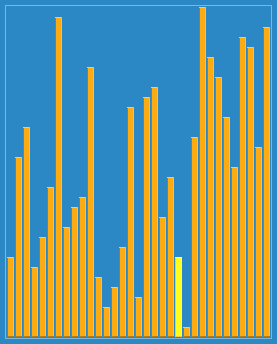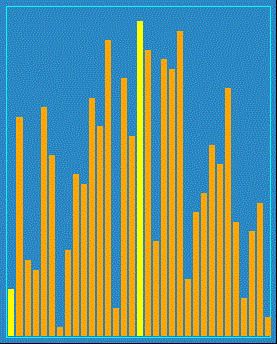Shell Sort is the unassuming, quiet type. The late-teens son of high-achieving Insertion Sort and Quick Sort, Shell Sort would much rather just curl up alone with a good sci-fi/fantasy book and sip hot cocoa.

He's a straight-A high school senior who really doesn't understand why he has to be at this stupid family reunion thing. In fact, he'd much rather be home right now, reading the latest N.K. Jemisin book.

Although, Auntie Heapy's cooking is pretty good. And the family football game isn't the worst thing in the universe. And as much as he's annoyed by younger brother Comb Sort, it's really nice to have him on the same team.

## Algorithm

1. DETERMINE the size of the gap we want to use, called h.
2. DIVIDE the main array into conceptual subarrays using elements that are h distance from each other (no concrete subarrays will actually be created).
3. SORT those subarrays in place.
4. REDUCE the gap size h so as to group items closer together for sorting.
5. CONTINUE until a pass has been done with h=1, when the list will be sorted.

## VisualizationThis is one of the visualizations I found for Shell Sort, but it's not very clear (at least to me) what's actually happening here, so let's see if we can break it down.

The first thing you need to know is that the goal of Shell Sort is to reduce the number of moves that must be made when gap size h = 1. In that situation, Shell Sort becomes Insertion Sort, and Insertion Sort does very poorly if the numbers to be sorted are far away from where they should be.

Imagine we have the following data:

a0 a1 a2 a3 a4 a5 a6 a7 a8 a9 a10 a11
43 18 91 6 29 62 30 81 79 52 7 51

Let's also say that our gap size h will be 5 for the first round of sorting. That means that Shell Sort will create the following theoretical subarrays:

{ a0, a5, a10 }
{ a1, a6, a11 }
{ a2, a7 }
{ a3, a8 }
{ a4, a9 }

Each of those subarrays will be sorted independently of one another, resulting in a collection that looks like this:

a0 a1 a2 a3 a4 a5 a6 a7 a8 a9 a10 a11
7 18 81 6 29 43 30 91 79 52 62 51

See how the 7 moved to the front of the array, much closer to where it should be when fully sorted?

Now let's shrink the gap size h to 3. Shell Sort will now use the following theoretical subarrays:

{ a0, a3, a6, a9 }
{ a1, a4, a7, a10 }
{ a2, a5, a8, a11 }

Sorting those subarrays results in a collection that looks like this:

a0 a1 a2 a3 a4 a5 a6 a7 a8 a9 a10 a11
6 18 43 7 29 51 30 62 79 52 91 81

Now the 6 is in front of the 7, and is in fact where it should be when the array is fully sorted. Remember that the goal here is not to fully sort the array, but to reduce the number of moves Insertion Sort must make in order to sort the array when h = 1. Speaking of, a pass through of this data during Insertion Sort results in, of course:

a0 a1 a2 a3 a4 a5 a6 a7 a8 a9 a10 a11
6 7 18 29 30 43 51 52 62 79 81 91

UPDATE (1 Aug 2019): Reader James Curran created another visualization of Shell Sort, and here it is:Finally, take a look at this "audibilization" of Shell Sort from YouTube:

## C# Implementation

``````class ShellSort
{
static int Sort(int[] array)
{
int length = array.Length;

for (int h = length / 2; h > 0; h /= 2)
{
for (int i = h; i < length; i += 1)
{
int temp = array[i];

int j;
for (j = i; j >= h && array[j - h] > temp; j -= h)
{
array[j] = array[j - h];
}

array[j] = temp;
}
}
return 0;
}

public static void Main()
{
int[] array = { 53, 19, 71, 3, 66, 62, 20, 84 };

Console.WriteLine("Shell Sort");

CommonFunctions.PrintInitial(array);

Sort(array);

CommonFunctions.PrintFinal(array);
}
}``````

## Time and Space Complexity

At first glance, it appears that Shell Sort actually performs worse than Insertion Sort, given that Insertion Sort's best case is O(n) and Shell Sort's best case is O(n log n). But in the real world the only time Insertion Sort performs in O(n) time is if the list is already sorted.

As stated earlier, Shell Sort's purpose is to minimize the distance items have to move when they are being sorted by the final stage where h = 1. The problem when trying to determine Shell Sort's time complexity is that such complexity depends entirely on what the implementation chooses for the values of h.

A final note: the implementation above actually has a time complexity of O(n2), and is used for simplicity and not fit for production.

## Summary

Shell Sort is mainly an improvement on Insertion Sort, by making long-distance moves less common when sorting the array. He'd much rather be home with a good book, but the family reunion isn't awful. Sometimes.

The next algorithm is the jock of the family, Comb Sort. He worries his life may not be going the way he planned.

Don't forget to check out the sample project over on GitHub!

Happy Coding!